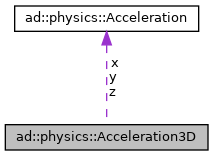DataType Acceleration3D. More...

`#include <ad/physics/Acceleration3D.hpp>`[legend]

## Public Types

typedef std::shared_ptr< Acceleration3DPtr
Smart pointer on Acceleration3D.

typedef std::shared_ptr< Acceleration3D const > ConstPtr
Smart pointer on constant Acceleration3D.

## Public Member Functions

Acceleration3D ()=default
standard constructor

~Acceleration3D ()=default
standard destructor

Acceleration3D (const Acceleration3D &other)=default
standard copy constructor

Acceleration3D (Acceleration3D &&other)=default
standard move constructor

Acceleration3Doperator= (const Acceleration3D &other)=default
standard assignment operator More...

Acceleration3Doperator= (Acceleration3D &&other)=default
standard move operator More...

bool operator== (const Acceleration3D &other) const
standard comparison operator More...

bool operator!= (const Acceleration3D &other) const
standard comparison operator More...

## Detailed Description

DataType Acceleration3D.

The rate of change of Velocity of an object with respect to time.

## ◆ operator!=()

 bool ad::physics::Acceleration3D::operator!= ( const Acceleration3D & other ) const
inline

standard comparison operator

Parameters
 [in] other Other Acceleration3D.
Returns
`true` if both Acceleration3D are different

## ◆ operator=() [1/2]

 Acceleration3D& ad::physics::Acceleration3D::operator= ( Acceleration3D && other )
default

standard move operator

Parameters
 [in] other Other Acceleration3D
Returns
Reference to this Acceleration3D.

## ◆ operator=() [2/2]

 Acceleration3D& ad::physics::Acceleration3D::operator= ( const Acceleration3D & other )
default

standard assignment operator

Parameters
 [in] other Other Acceleration3D
Returns
Reference to this Acceleration3D.

## ◆ operator==()

 bool ad::physics::Acceleration3D::operator== ( const Acceleration3D & other ) const
inline

standard comparison operator

Parameters
 [in] other Other Acceleration3D
Returns
`true` if both Acceleration3D are equal

## ◆ x

The x component of the acceleration